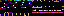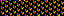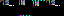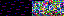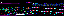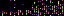```from array import array

from pygame.mixer import Sound, get_init

class Samples(Sound):

def __init__(self):
self.set_amplitude()
Sound.__init__(self, self.build())

def set_amplitude(self):
self.amplitude = (1 << (self.get_sample_width() * 8 - 1)) - 1

def get_sample_width(self):
return abs(get_init() / 8)

def build(self):
pass

def get_empty_array(self, length):
return array(self.get_array_typecode(),  * length)

def get_array_typecode(self):
return [None, "b", "h"][self.get_sample_width()]
```
```from pgfw.Setup import Setup

if __name__ == "__main__":
Setup().setup()
```
```from pgfw.SetupWin import SetupWin

if __name__ == "__main__":
SetupWin().setup()
```
```use feature "say";

package Song;

sub new
{
my \$class = shift;
my \$self = bless {frame_duration => \$ARGV, step => \$ARGV}, \$class;
\$self;
}

{
my \$self = shift;
\$self->buildFrames();
}

{
my \$self = shift;
my \$background = Image::Magick->new;
\$self->{background} = \$background;
}

{
my \$self = shift;
}

{
my \$self = shift;
my \$border = Image::Magick->new;
\$self->{border} = \$border;
}

{
my \$self = shift;
}

sub buildFrames
{
my \$self = shift;
my @frames;
my \$strip_width = \$strip->Get("width");
my \$background = \$self->{background};
my \$background_width = \$background->Get("width");
my \$background_height = \$background->Get("height");
my \$mask_size = \$background_width . "x" . \$background_height;
my \$points = [0, 0, 0, 0,
\$background_width, 0, \$background_width, 0,
0, \$background_height, 3, \$background_height - 2,
\$background_width, \$background_height, \$background_width - 3, \$background_height - 2];
my \$x = 0;
while (\$x > -\$strip_width)
{
my \$frame = \$background->Clone();
if (\$strip_width + \$x < \$background_width)
{
}
\$frame->Composite(image=>\$self->{border});
\$frame->Distort(method=>"Perspective", points=>\$points,
"virtual-pixel"=>"transparent");
\$frame->Trim();
push @frames, \$frame;
\$x -= \$self->{step};
}
\$self->{frames} = \@frames;
}

sub write
{
my \$self = shift;
\$directory = "new/";
mkdir \$directory;
my \$frames = \$self->{frames};
my \$index;
for (0..@{\$frames} - 1)
{
\$index = sprintf("%02d", \$_);
\$name = \$directory . \$index . "-" . \$self->{frame_duration} . ".gif";
@{\$frames}[\$_]->Write(\$name);
\$self->convertToPNG(\$name);
}
}

sub convertToPNG
{
my \$self = shift;
my \$name = shift;
my \$img = Image::Magick->new;
\$name =~ s/gif/png/;
\$img->Write(\$name);
}

1;
```
```#!/usr/bin/perl

use strict;
use warnings;

use feature "say";

use Data::Dumper;
use CGI;

require "writeAnimation.pl";

generate();

sub generate
{
my \$cgi = CGI->new;
my \$params = \$cgi->Vars;
say "<pre>";
if (!\$params->{"type"})
{
say Dumper(\$params);
say "No image type specified";
}
else
{
writeAnimation(\$params);
}
say "</pre>";
}
```
```use strict;
use warnings;
use feature "say";

use Data::Dumper;
use Animation::Imitation;

sub writeAnimation
{
my \$arguments = shift;
my \$animation;
for (\$arguments->{"type"})
{
if (/^im.*/ || /^1\$/)
{
\$animation = Animation::Imitation->new(\$arguments);
}
}
\$animation->write();
}

1;
```
35.175.107.142
35.175.107.142
35.175.107.142

June 23, 2019